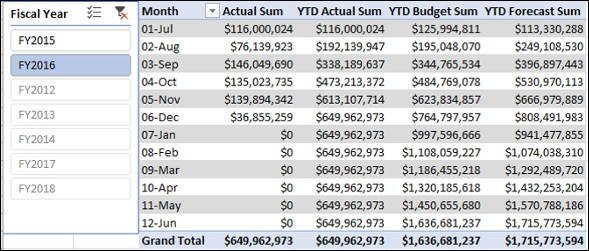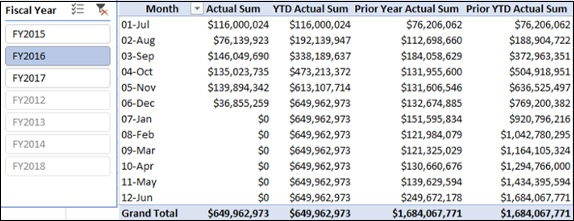# Year-to-Date Measures and Analysis

To calculate a result that includes a starting balance from the beginning of a period, such as a fiscal year, up to a specific period in time, you can use DAX Time Intelligence functions. This will enable you to analyze data on a month level.

In this chapter, you will learn how to create Year-to-Date measures and how to carry out data analysis with the same.

### Creating Year-to-Date Actual Sum Measure

Create Year-to-Date Actual Sum measure as follows −

YTD Actual Sum:=TOTALYTD([Actual Sum], 'Date'[Date], ALL('Date'), "6/30")

### Creating Year-to-Date Budget Sum Measure

Create Year-to-Date Budget Sum measure as follows −

YTD Budget Sum:=TOTALYTD([Budget Sum], 'Date'[Date], ALL('Date'), "6/30")

### Creating Year-to-Date Forecast Sum Measure

Create Year-to-Date Forecast Sum measure as follows −

YTD Forecast Sum:=TOTALYTD([Forecast Sum], 'Date'[Date], ALL('Date'), "6/30")

### Creating Prior Year-to-Date Actual Sum Measure

Create Prior Year-to-Date Actual Sum measure as follows −

Prior YTD Actual Sum:=TOTALYTD([Prior Year Actual Sum], 'Date'[Date], ALL('Date'), "6/30")

## Analyzing Data with Year-to-Date Measures

Create a Power PivotTable as follows −

• Add Month from Date table to Rows.

• Add the measures Actual Sum, YTD Actual Sum, YTD Budget Sum, and YTD Forecast Sum from the Finance Data table to Values.

• Insert a Slicer on the Fiscal Year from the Date table.

• Select FY2016 in the Slicer.Create a Power PivotTable as follows −

• Add Month from Date table to Rows.

• Add the measures Actual Sum, YTD Actual Sum, Prior Year Actual Sum, and Prior Year YTD Actual Sum from the Finance Data table to Values.

• Insert a Slicer on the Fiscal Year from the Date table.

• Select FY2016 in the Slicer.